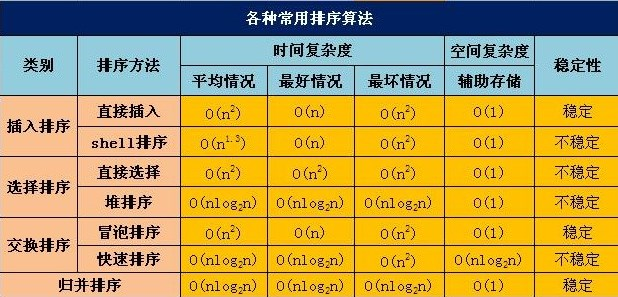# 冒泡排序

### 基本思想:

Java实现

``````public static void bubbleSort(int[] numbers) {
int temp = 0;
int size = numbers.length;
boolean flag = true;
for (int i = 0; i < size - 1&&flag; i++) {
flag = false;
for (int j = 0; j < size - 1 - i; j++) {
if (numbers[j] > numbers[j + 1]) // 交换两数位置
{
temp = numbers[j];
numbers[j] = numbers[j + 1];
numbers[j + 1] = temp;
flag = true;
}
}
}
}``````

# 选择排序算法

### 基本思想：

Java 实现

``````public static void selectSort(int[] numbers) {
int size = numbers.length; // 数组长度
int temp = 0; // 中间变量
for (int i = 0; i < size-1; i++) {
int k = i; // 待确定的位置
// 选择出应该在第i个位置的数
for (int j = size - 1; j > i; j--) {
if (numbers[j] < numbers[k]) {
k = j;
}
}
// 交换两个数
temp = numbers[i];
numbers[i] = numbers[k];
numbers[k] = temp;
}
}``````

# 插入排序算法

### 基本思想：

Java 实现

``````public static void insertSort(int[] numbers) {
int size = numbers.length;
int temp = 0;
int j = 0;
for (int i = 1; i < size; i++) {
temp = numbers[i];
// 假如temp比前面的值小，则将前面的值后移
for (j = i; j > 0 && temp < numbers[j - 1]; j--) {
numbers[j] = numbers[j - 1];
}
numbers[j] = temp;
}
}``````

O(n*n) 性能上优于冒泡排序和选择排序

# 希尔排序算法

### 基本思想：

Java 实现

``````/**
* 希尔排序的原理:根据需求，如果你想要结果从小到大排列，它会首先将数组进行分组，然后将较小值移到前面，较大值
* 移到后面，最后将整个数组进行插入排序，这样比起一开始就用插入排序减少了数据交换和移动的次数，
* 可以说希尔排序是加强 版的插入排序 拿数组5, 2,8, 9, 1, 3，4来说，数组长度为7，当increment为3时，数组分为两个序列
* 5，2，8和9，1，3，4，第一次排序，9和5比较，1和2比较，3和8比较，4和比其下标值小increment的数组值相比较
* 此例子是按照从小到大排列，所以小的会排在前面，第一次排序后数组为5, 1, 3, 4, 2, 8，9
* 第一次后increment的值变为3/2=1,此时对数组进行插入排序， 实现数组从大到小排
*/
public static void shellSort(int[] data) {
int j = 0;
int temp = 0;
// 每次将步长缩短为原来的一半
for (int increment = data.length / 2; increment > 0; increment /= 2) {
for (int i = increment; i < data.length; i++) {
temp = data[i];
for (j = i; j >= increment; j -= increment) {
if (temp < data[j - increment])// 从小到大排
{
data[j] = data[j - increment];
} else {
break;
}
}
data[j] = temp;
}
}``````

# 堆排序算法

### 基本思想:

Java 实现

``````public static void heapSort(int[] a){
int arrayLength = a.length;
// 循环建堆
for (int i = 0; i < arrayLength - 1; i++) {
// 建堆
buildMaxHeap(a, arrayLength - 1 - i);
// 交换堆顶和最后一个元素
swap(a, 0, arrayLength - 1 - i);
System.out.println(Arrays.toString(a));
}
}
// 对data数组从0到lastIndex建大顶堆
public static void buildMaxHeap(int[] data, int lastIndex) {
// 从lastIndex处节点（最后一个节点）的父节点开始
for (int i = (lastIndex - 1) / 2; i >= 0; i--) {
// k保存正在判断的节点
int k = i;
// 如果当前k节点的子节点存在
while (k * 2 + 1 <= lastIndex) {
// k节点的左子节点的索引
int biggerIndex = 2 * k + 1;
// 如果biggerIndex小于lastIndex，即biggerIndex+1代表的k节点的右子节点存在
if (biggerIndex < lastIndex) {
// 若果右子节点的值较大
if (data[biggerIndex] < data[biggerIndex + 1]) {
// biggerIndex总是记录较大子节点的索引
biggerIndex++;
}
}
// 如果k节点的值小于其较大的子节点的值
if (data[k] < data[biggerIndex]) {
// 交换他们
swap(data, k, biggerIndex);
// 将biggerIndex赋予k，开始while循环的下一次循环，重新保证k节点的值大于其左右子节点的值
k = biggerIndex;
} else {
break;
}
}
}
}
// 交换
private static void swap(int[] data, int i, int j) {
int tmp = data[i];
data[i] = data[j];
data[j] = tmp;
}``````

# 快速排序算法

### 基本思想：

Java 实现

``````/**
* 快速排序
*
* @param numbers
*            带排序数组
*/
public static void quick(int[] numbers) {
if (numbers.length > 0) // 查看数组是否为空
{
quickSort(numbers, 0, numbers.length - 1);
}
}
/**
*
* @param numbers
*            带排序数组
* @param low
*            开始位置
* @param high
*            结束位置
*/
public static void quickSort(int[] numbers, int low, int high) {
if (low >= high) {
return;
}
int middle = getMiddle(numbers, low, high); // 将numbers数组进行一分为二
quickSort(numbers, low, middle - 1); // 对低字段表进行递归排序
quickSort(numbers, middle + 1, high); // 对高字段表进行递归排序
}
/**
* 查找出中轴（默认是最低位low）的在numbers数组排序后所在位置
*
* @param numbers
*            带查找数组
* @param low
*            开始位置
* @param high
*            结束位置
* @return 中轴所在位置
*/
public static int getMiddle(int[] numbers, int low, int high) {
int temp = numbers[low]; // 数组的第一个作为中轴
while (low < high) {
while (low < high && numbers[high] > temp) {
high--;
}
numbers[low] = numbers[high];// 比中轴小的记录移到低端
while (low < high && numbers[low] < temp) {
low++;
}
numbers[high] = numbers[low]; // 比中轴大的记录移到高端
}
numbers[low] = temp; // 中轴记录到尾
return low; // 返回中轴的位置
}``````

# 归并排序算法

### 基本思想:

Java 实现

``````/**
* 归并排序
* 简介:将两个（或两个以上）有序表合并成一个新的有序表 即把待排序序列分为若干个子序列，每个子序列是有序的。然后再把有序子序列合并为整体有序序列
* 时间复杂度为O(nlogn)
* 稳定排序方式
* @param nums 待排序数组
* @return 输出有序数组
*/
public static int[] sort(int[] nums, int low, int high) {
int mid = (low + high) / 2;
if (low < high) {
// 左边
sort(nums, low, mid);
// 右边
sort(nums, mid + 1, high);
// 左右归并
merge(nums, low, mid, high);
}
return nums;
}
/**
* 将数组中low到high位置的数进行排序
* @param nums 待排序数组
* @param low 待排的开始位置
* @param mid 待排中间位置
* @param high 待排结束位置
*/
public static void merge(int[] nums, int low, int mid, int high) {
int[] temp = new int[high - low + 1];
int i = low;// 左指针
int j = mid + 1;// 右指针
int k = 0;
// 把较小的数先移到新数组中
while (i <= mid && j <= high) {
if (nums[i] < nums[j]) {
temp[k++] = nums[i++];
} else {
temp[k++] = nums[j++];
}
}
// 把左边剩余的数移入数组
while (i <= mid) {
temp[k++] = nums[i++];
}
// 把右边边剩余的数移入数组
while (j <= high) {
temp[k++] = nums[j++];
}
// 把新数组中的数覆盖nums数组
for (int k2 = 0; k2 < temp.length; k2++) {
nums[k2 + low] = temp[k2];
}
}``````

# 各种算法的时间复杂度等性能比较